# Unicus Olympiads - UCTO PDF Sample Papers for Class 3

Class 3 sample paper & practice questions for Unicus Critical Thinking Olympiad (UCTO) PDF Sample Papers are given below. Syllabus for UCTO is also mentioned for these exams. You can refer these sample paper & quiz for preparing for the UCTO exam.#### Resources:

##### Sample Questions from Olympiad Success:
 Q.1 Q.2 Q.3 Q.4 Q.5 Q.6 Q.7 Q.8 Q.9 Q.10
 Q.1 How many slanting lines are there in the given lines?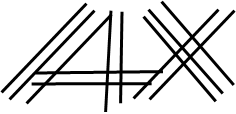a) 10 b) 7 c) 9 d) 8
 Q.2 If January = 31, February = 28, then March = ? a) 31 b) 30 c) 29 d) 27
 Q.3 Find the next figure in the following pattern: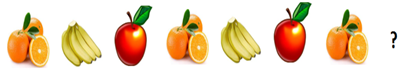a)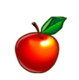b)c)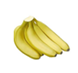d)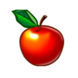Q.4 Three of given figures are identical in a certain way. Choose the one that is different from the rest: a)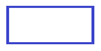b)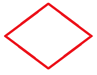c)d)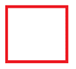Q.5 Choose the odd one out: a)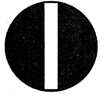b)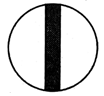c)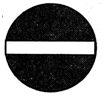d)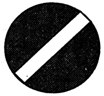Q.6 From the following options, select the one which is embedded in the question figure (size may differ):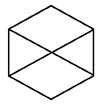a)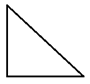b)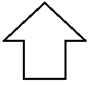c)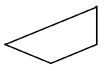d)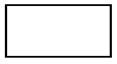Q.7 Which is the odd one out? a)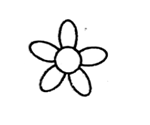b)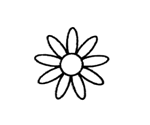c)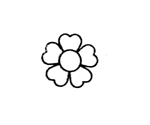d)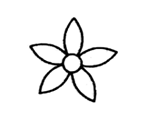Q.8 Among the 10 students, Rekha stood 5th. If the sum of positions of Rekha and Ajit is 12, what is Ajit's position? a) 8 b) 3 c) 7 d) 4
 Q.9 There are __________ rectangles and __________ circles in the following figure.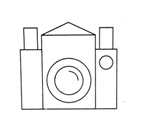a) 6, 2 b) 7, 3 c) 4, 2 d) 5, 3
 Q.10 Which among the following can you group with the given figure?a)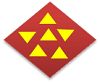b)c)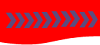d)Sample PDF of Unicus Olympiads - Unicus Critical Thinking Olympiad (UCTO) PDF Sample Papers for Class 3:

 Q.1 )d Q.2 )a Q.3 )c Q.4 )c Q.5 )b Q.6 )c Q.7 )b Q.8 )c Q.9 )d Q.10 )a

Q.1 : d | Q.2 : a | Q.3 : c | Q.4 : c | Q.5 : b | Q.6 : c | Q.7 : b | Q.8 : c | Q.9 : d | Q.10 : a